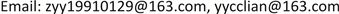﻿ 一种新的股票模型的期权定价公式 Option Pricing Formula for a New Stock Model

Vol. 07  No. 10 ( 2018 ), Article ID: 27207 , 8 pages
10.12677/AAM.2018.710142

Option Pricing Formula for a New Stock Model

Yuanyuan Zhang, Cuilian You

College of Mathematics and Information Science, Hebei University, Baoding HebeiReceived: Oct. 1st, 2018; accepted: Oct. 15th, 2018; published: Oct. 22nd, 2018ABSTRACT

The option pricing problem is one of central contents in modern finance. Black and Scholes assume that the fluctuation of stock price conforms to geometric Brownian motion, and then establishes stochastic financial mathematics on this basis. However, the uncertainties in the uncertain environment are not only random but also fuzzy, so it is necessary to study the fuzzy financial market. Based on the credibility theory, this paper discusses the case that the execution price of an option satisfies a diffusion process, and obtains the option pricing formula of this new stock model.

Keywords:Fuzzy Process, Liu Process, Stock Model, Fuzzy Differential Equation, Option Pricing1. 引言

Liu和Liu  在2002年提出了可信性测度的概念。之后，Li和Liu  给出了可信性测度的充要条件。Liu  在2004年建立了可信性理论，之后Liu  在2007年完善了这一理论，随后这一理论成为了研究模糊现象的一个数学分支。为了描述动态模糊现象，Liu  提出了模糊环境下布朗运动的对应概念——Liu过程，并给出Liu微分定义和Liu积分的定义，它们分别对应Ito微分和Ito积分。有关Liu过程方面，学者们做了大量的研究，You，Huo和Wang  将Liu过程，Liu积分，Liu公式推广到多维情形。You和Wang  介绍了模糊积分存在的三个条件和三类模糊积分性质。You，Ma和Huo  给出了一类广义Liu积分的定义，并且还得到了这种积分的性质和判别定理   。此外，Dai证明了Liu过程是Lipschitz连续且具有有限变差。Kaleva  ，Kaleva  和Ding，Ma和Kandel  对传统的模糊微分方程进行了研究。这些模糊微分方程实际上是带有模糊参数的微分方程。Liu  定义了一种新的模糊微分方程：

$\text{d}{X}_{t}=f\left(t,{X}_{t}\right)\text{d}t+g\left(t,{X}_{t}\right)\text{d}{C}_{t},$

2. 预备知识

$\text{Cr}\left\{\xi \in B\right\}=\frac{1}{2}\left(\underset{x\in B}{\mathrm{sup}}\mu \left(x\right)+1-\underset{x\in {B}^{c}}{\mathrm{sup}}\mu \left(x\right)\right).$

$\text{Cr}\left\{\underset{i=1}{\overset{m}{\cap }}\left\{{\xi }_{i}\in {B}_{i}\right\}\right\}=\underset{1\le i\le m}{\mathrm{min}}\text{Cr}\left\{{\xi }_{i}\in {B}_{i}\right\},$

${X}_{{t}_{1}}-{X}_{{t}_{0}},{X}_{{t}_{2}}-{X}_{{t}_{1}},\cdots ,{X}_{{t}_{k}}-{X}_{{t}_{k-1}}$

1) ${C}_{0}=0$

2) ${C}_{t}$ 具有平稳独立增量，

3) 任意增量 ${C}_{t+s}-{C}_{s}$ 是正态分布模糊变量，即期望为 $et$ ，方差为 ${\delta }^{2}{t}^{2}$ ，隶属函数为

$\mu \left(x\right)=2{\left(1+\mathrm{exp}\left(\frac{\pi |x-et|}{\sqrt{6}\delta t}\right)\right)}^{-1},x\in \Re ,$

${X}_{t}=\mathrm{exp}\left(et+\delta {C}_{t}\right)$

$\text{d}{X}_{t}=\frac{\partial h}{\partial t}\left(t,{C}_{t}\right)\text{d}t+\frac{\partial h}{\partial c}\left(t,{C}_{t}\right)\text{d}{C}_{t}.$

$\left\{\begin{array}{l}{B}_{t}={B}_{0}\mathrm{exp}\left(rt\right)\hfill \\ {S}_{t}={S}_{0}\mathrm{exp}\left(et+\delta {C}_{t}\right)\hfill \end{array}$

$\left\{\begin{array}{l}\text{d}{B}_{t}=r{B}_{t}\text{d}t\hfill \\ \text{d}{S}_{t}=e{S}_{t}\text{d}t+\delta {S}_{t}\text{d}{C}_{t}\hfill \end{array}$

3. 一种新的模糊股票模型

$\left\{\begin{array}{l}\text{d}{B}_{t}=r{B}_{t}\text{d}t\hfill \\ \text{d}{S}_{t}={e}_{1}{S}_{t}\text{d}t+{\delta }_{1}{S}_{t}\text{d}{C}_{1t}\hfill \\ \text{d}{Y}_{t}={e}_{2}{Y}_{t}\text{d}t+{\delta }_{2}{Y}_{t}\text{d}{C}_{2t}\hfill \end{array}$ (3.1)

4. 欧式看涨期权定价公式

$\mathrm{exp}\left(-rT\right){\left({S}_{T}-{Y}_{T}\right)}^{+}.$

$\begin{array}{l}f\left({S}_{0},{Y}_{0},{e}_{1},{e}_{2},{\delta }_{1},{\delta }_{2},r\right)\\ =\mathrm{exp}\left(-rT\right)E\left[{S}_{0}\mathrm{exp}\left({e}_{1}T+{\delta }_{1}{C}_{1T}\right)-{Y}_{0}\mathrm{exp}{\left({e}_{2}T+{\delta }_{2}{C}_{2T}\right)}^{+}\right].\end{array}$ (4.1)

$\begin{array}{l}f\left({S}_{0},{Y}_{0},{e}_{1},{e}_{2},{\delta }_{1},{\delta }_{2},r\right)\\ ={S}_{0}\mathrm{exp}\left(\left({e}_{1}-r\right)T\right){\int }_{0}^{+\infty }{\left(1+{x}^{\pi /\sqrt{6}{\delta }_{1}T}\right)}^{-1}\text{d}x\\ \text{\hspace{0.17em}}\text{\hspace{0.17em}}\text{\hspace{0.17em}}-{Y}_{0}\mathrm{exp}\left(\left({e}_{2}-r\right)T\right){\int }_{0}^{+\infty }{\left(1+{x}^{\pi /\sqrt{6}{\delta }_{2}T}\right)}^{-1}\text{d}x.\end{array}$

$\begin{array}{l}f\left({S}_{0},{Y}_{0},{e}_{1},{e}_{2},{\delta }_{1},{\delta }_{2},r\right)\\ =\mathrm{exp}\left(-rT\right)E\left[{S}_{0}\mathrm{exp}\left({e}_{1}T+{\delta }_{1}{C}_{1T}\right)-{Y}_{0}\mathrm{exp}{\left({e}_{2}T+{\delta }_{2}{C}_{2T}\right)}^{+}\right]\\ =\mathrm{exp}\left(-rT\right)\left\{E\left[{S}_{0}\mathrm{exp}\left({e}_{1}T+{\delta }_{1}{C}_{1T}\right)-E\left[{Y}_{0}\mathrm{exp}\left({e}_{2}T+{\delta }_{2}{C}_{2T}\right)\right]\right]\right\}\\ ={S}_{0}\mathrm{exp}\left(-rT\right){\int }_{0}^{+\infty }\text{Cr}\left\{\mathrm{exp}\left({e}_{1}T+{\delta }_{1}{C}_{1T}\right)\ge x\right\}\text{d}x\\ \text{\hspace{0.17em}}\text{\hspace{0.17em}}\text{\hspace{0.17em}}-{Y}_{0}\mathrm{exp}\left(-rT\right){\int }_{0}^{+\infty }\text{Cr}\left\{\mathrm{exp}\left({e}_{2}T+{\delta }_{2}{C}_{2T}\right)\ge x\right\}\text{d}x\end{array}$

$\begin{array}{l}={S}_{0}\mathrm{exp}\left(-rT\right){\int }_{0}^{+\infty }\text{Cr}\left\{{C}_{1T}\ge \frac{\mathrm{ln}x-{e}_{1}T}{{\delta }_{1}}\right\}\text{d}x\\ \text{\hspace{0.17em}}\text{\hspace{0.17em}}\text{\hspace{0.17em}}-{Y}_{0}\mathrm{exp}\left(-rT\right){\int }_{0}^{+\infty }\text{Cr}\left\{{C}_{2T}\ge \frac{\mathrm{ln}x-{e}_{2}T}{{\delta }_{2}}\right\}\text{d}x\\ ={S}_{0}\mathrm{exp}\left(-rT\right){\int }_{0}^{\mathrm{exp}\left({e}_{1}T\right)}\left[1-\frac{1}{2}\underset{y<\frac{\mathrm{ln}x-{e}_{1}T}{{\delta }_{1}}}{\mathrm{sup}}u\left(y\right)\right]\text{d}x\\ \text{\hspace{0.17em}}\text{\hspace{0.17em}}\text{\hspace{0.17em}}+{S}_{0}\mathrm{exp}\left(-rT\right){\int }_{\mathrm{exp}\left({e}_{1}T\right)}^{+\infty }\left[\frac{1}{2}\underset{y\ge \frac{\mathrm{ln}x-{e}_{1}T}{{\delta }_{1}}}{\mathrm{sup}}u\left(y\right)\right]\text{d}x\\ \text{\hspace{0.17em}}\text{\hspace{0.17em}}\text{\hspace{0.17em}}-{Y}_{0}\mathrm{exp}\left(-rT\right){\int }_{0}^{\mathrm{exp}\left({e}_{2}T\right)}\left[1-\frac{1}{2}\underset{y<\frac{\mathrm{ln}x-{e}_{2}T}{{\delta }_{2}}}{\mathrm{sup}}u\left(y\right)\right]\text{d}x\end{array}$

$\begin{array}{l}\text{\hspace{0.17em}}\text{\hspace{0.17em}}\text{\hspace{0.17em}}-{Y}_{0}\mathrm{exp}\left(-rT\right){\int }_{\mathrm{exp}\left({e}_{2}T\right)}^{+\infty }\left[\frac{1}{2}\underset{y\ge \frac{\mathrm{ln}x-{e}_{2}T}{{\delta }_{2}}}{\mathrm{sup}}u\left(y\right)\right]\text{d}x\\ ={S}_{0}\mathrm{exp}\left(-rT\right){\int }_{0}^{\mathrm{exp}\left({e}_{1}T\right)}1-{\left(1+\mathrm{exp}\left(\frac{\pi \left({e}_{1}T-\mathrm{ln}x\right)}{\sqrt{6}{\delta }_{1}T}\right)\right)}^{-1}\text{d}x\\ \text{\hspace{0.17em}}\text{\hspace{0.17em}}\text{\hspace{0.17em}}+{S}_{0}\mathrm{exp}\left(-rT\right){\int }_{\mathrm{exp}\left({e}_{1}T\right)}^{+\infty }{\left(1+\mathrm{exp}\left(\frac{\pi \left(\mathrm{ln}x-{e}_{1}T\right)}{\sqrt{6}{\delta }_{1}T}\right)\right)}^{-1}\text{d}x\\ \text{\hspace{0.17em}}\text{\hspace{0.17em}}\text{\hspace{0.17em}}-{Y}_{0}\mathrm{exp}\left(-rT\right){\int }_{0}^{\mathrm{exp}\left({e}_{2}T\right)}1-{\left(1+\mathrm{exp}\left(\frac{\pi \left({e}_{2}T-\mathrm{ln}x\right)}{\sqrt{6}{\delta }_{2}T}\right)\right)}^{-1}\text{d}x\\ \text{\hspace{0.17em}}\text{\hspace{0.17em}}\text{\hspace{0.17em}}-{Y}_{0}\mathrm{exp}\left(-rT\right){\int }_{\mathrm{exp}\left({e}_{2}T\right)}^{+\infty }{\left(1+\mathrm{exp}\left(\frac{\pi \left(\mathrm{ln}x-{e}_{2}T\right)}{\sqrt{6}{\delta }_{2}T}\right)\right)}^{-1}\text{d}x\end{array}$

$\begin{array}{l}={S}_{0}\mathrm{exp}\left(-rT\right){\int }_{0}^{+\infty }\frac{\mathrm{exp}\left(\pi {e}_{1}/\sqrt{6}{\delta }_{1}T\right)}{\mathrm{exp}\left(\pi {e}_{1}/\sqrt{6}{\delta }_{1}T\right)+{x}^{\pi /\sqrt{6}{\delta }_{1}T}}\text{d}x\\ \text{\hspace{0.17em}}\text{\hspace{0.17em}}\text{\hspace{0.17em}}-{Y}_{0}\mathrm{exp}\left(-rT\right){\int }_{0}^{+\infty }\frac{\mathrm{exp}\left(\pi {e}_{2}/\sqrt{6}{\delta }_{2}T\right)}{\mathrm{exp}\left(\pi {e}_{2}/\sqrt{6}{\delta }_{2}T\right)+{x}^{\pi /\sqrt{6}{\delta }_{2}T}}\text{d}x\\ ={S}_{0}\mathrm{exp}\left(\left({e}_{1}-r\right)T\right){\int }_{0}^{+\infty }{\left(1+{x}^{\pi /\sqrt{6}{\delta }_{1}T}\right)}^{-1}\text{d}x\\ \text{\hspace{0.17em}}\text{\hspace{0.17em}}\text{\hspace{0.17em}}-{Y}_{0}\mathrm{exp}\left(\left({e}_{2}-r\right)T\right){\int }_{0}^{+\infty }{\left(1+{x}^{\pi /\sqrt{6}{\delta }_{2}T}\right)}^{-1}\text{d}x\end{array}$

$\begin{array}{l}syms\text{ }x;\\ y=\text{'}30*\mathrm{exp}\left(\left(0.06-0.08\right)*0.25\right)./\left(1+x^\left(pi./sqrt\left(6\right)*0.25*0.25\right)\right)\right)\\ \text{ }\text{\hspace{0.17em}}\text{\hspace{0.17em}}-28*\mathrm{exp}\left(\left(0.05-0.08\right)*0.25\right)./\left(1+x^\left(pi./sqrt\left(6\right)*0.2*0.25\right)\right)\right)\text{'};\\ f=quad\left(y,0,100\right)\end{array}$

5. 欧式看跌期权定价公式

$\begin{array}{l}f\left({S}_{0},{Y}_{0},{e}_{1},{e}_{2},{\delta }_{1},{\delta }_{2},r\right)\\ =\mathrm{exp}\left(-rT\right)E\left[{Y}_{0}\mathrm{exp}\left({e}_{2}T+{\delta }_{2}{C}_{2T}\right)-{S}_{0}\mathrm{exp}{\left({e}_{1}T+{\delta }_{1}{C}_{1T}\right)}^{+}\right].\end{array}$ (4.2)

$\begin{array}{l}f\left({S}_{0},{Y}_{0},{e}_{1},{e}_{2},{\delta }_{1},{\delta }_{2},r\right)\\ ={Y}_{0}\mathrm{exp}\left(\left({e}_{2}-r\right)T\right){\int }_{0}^{+\infty }{\left(1+{x}^{\pi /\sqrt{6}{\delta }_{2}T}\right)}^{-1}\text{d}x\\ \text{\hspace{0.17em}}\text{\hspace{0.17em}}\text{\hspace{0.17em}}-{S}_{0}\mathrm{exp}\left(\left({e}_{1}-r\right)T\right){\int }_{0}^{+\infty }{\left(1+{x}^{\pi /\sqrt{6}{\delta }_{1}T}\right)}^{-1}\text{d}x.\end{array}$

$\begin{array}{l}f\left({S}_{0},{Y}_{0},{e}_{1},{e}_{2},{\delta }_{1},{\delta }_{2},r\right)\\ =\mathrm{exp}\left(-rT\right)E\left[{Y}_{0}\mathrm{exp}\left({e}_{2}T+{\delta }_{2}{C}_{2T}\right)-{S}_{0}\mathrm{exp}{\left({e}_{1}T+{\delta }_{1}{C}_{1T}\right)}^{+}\right]\\ =\mathrm{exp}\left(-rT\right)\left\{E\left[{Y}_{0}\mathrm{exp}\left({e}_{2}T+{\delta }_{2}{C}_{2T}\right)\right]-E\left[{S}_{0}\mathrm{exp}\left({e}_{1}T+{\delta }_{\text{1}}{C}_{1T}\right)\right]\right\}\\ ={Y}_{0}\mathrm{exp}\left(-rT\right){\int }_{0}^{+\infty }\text{Cr}\left\{\mathrm{exp}\left({e}_{2}T+{\delta }_{2}{C}_{2T}\right)\ge x\right\}\text{d}x\\ \text{\hspace{0.17em}}\text{\hspace{0.17em}}\text{\hspace{0.17em}}-{S}_{0}\mathrm{exp}\left(-rT\right){\int }_{0}^{+\infty }\text{Cr}\left\{\mathrm{exp}\left({e}_{1}T+{\delta }_{1}{C}_{1T}\right)\ge x\right\}\text{d}x\end{array}$

$\begin{array}{l}={Y}_{0}\mathrm{exp}\left(-rT\right){\int }_{0}^{+\infty }\text{Cr}\left\{{C}_{2T}\ge \frac{\mathrm{ln}x-{e}_{2}T}{{\delta }_{2}}\right\}\text{d}x\\ \text{\hspace{0.17em}}\text{\hspace{0.17em}}\text{\hspace{0.17em}}-{S}_{0}\mathrm{exp}\left(-rT\right){\int }_{0}^{+\infty }\text{Cr}\left\{{C}_{1T}\ge \frac{\mathrm{ln}x-{e}_{1}T}{{\delta }_{1}}\right\}\text{d}x\\ ={Y}_{0}\mathrm{exp}\left(-rT\right){\int }_{0}^{\mathrm{exp}\left({e}_{2}T\right)}\left[1-\frac{1}{2}\underset{y<\frac{\mathrm{ln}x-{e}_{2}T}{{\delta }_{2}}}{\mathrm{sup}}u\left(y\right)\right]\text{d}x\\ \text{\hspace{0.17em}}\text{\hspace{0.17em}}\text{\hspace{0.17em}}+{Y}_{0}\mathrm{exp}\left(-rT\right){\int }_{\mathrm{exp}\left({e}_{2}T\right)}^{+\infty }\left[\frac{1}{2}\underset{y\ge \frac{\mathrm{ln}x-{e}_{2}T}{{\delta }_{2}}}{\mathrm{sup}}u\left(y\right)\right]\text{d}x\\ \text{\hspace{0.17em}}\text{\hspace{0.17em}}\text{\hspace{0.17em}}-{S}_{0}\mathrm{exp}\left(-rT\right){\int }_{0}^{\mathrm{exp}\left({e}_{1}T\right)}\left[1-\frac{1}{2}\underset{y<\frac{\mathrm{ln}x-{e}_{1}T}{{\delta }_{1}}}{\mathrm{sup}}u\left(y\right)\right]\text{d}x\end{array}$

$\begin{array}{l}\text{\hspace{0.17em}}\text{\hspace{0.17em}}\text{\hspace{0.17em}}-{S}_{0}\mathrm{exp}\left(-rT\right){\int }_{\mathrm{exp}\left({e}_{1}T\right)}^{+\infty }\left[\frac{1}{2}\underset{y\ge \frac{\mathrm{ln}x-{e}_{1}T}{{\delta }_{1}}}{\mathrm{sup}}u\left(y\right)\right]\text{d}x\\ ={Y}_{0}\mathrm{exp}\left(-rT\right){\int }_{0}^{+\infty }\frac{\mathrm{exp}\left(\pi {e}_{2}/\sqrt{6}{\delta }_{2}T\right)}{\mathrm{exp}\left(\pi {e}_{2}/\sqrt{6}{\delta }_{2}T\right)+{x}^{\pi /\sqrt{6}{\delta }_{2}T}}\text{d}x\\ \text{\hspace{0.17em}}\text{\hspace{0.17em}}\text{\hspace{0.17em}}-{S}_{0}\mathrm{exp}\left(-rT\right){\int }_{0}^{+\infty }\frac{\mathrm{exp}\left(\pi {e}_{1}/\sqrt{6}{\delta }_{1}T\right)}{\mathrm{exp}\left(\pi {e}_{1}/\sqrt{6}{\delta }_{1}T\right)+{x}^{\pi /\sqrt{6}{\delta }_{1}T}}\text{d}x\\ ={Y}_{0}\mathrm{exp}\left(\left({e}_{2}-r\right)T\right){\int }_{0}^{+\infty }{\left(1+{x}^{\pi /\sqrt{6}{\delta }_{2}T}\right)}^{-1}\text{d}x\\ \text{\hspace{0.17em}}\text{\hspace{0.17em}}\text{\hspace{0.17em}}-{S}_{0}\mathrm{exp}\left(\left({e}_{1}-r\right)T\right){\int }_{0}^{+\infty }{\left(1+{x}^{\pi /\sqrt{6}{\delta }_{1}T}\right)}^{-1}\text{d}x.\end{array}$

$\begin{array}{l}syms\text{ }x;\\ y=\text{'}32*\mathrm{exp}\left(\left(0.05-0.08\right)*0.25\right)./\left(1+x^\left(pi./sqrt\left(6\right)*0.2*0.25\right)\right)\right)\\ \text{ }\text{\hspace{0.17em}}\text{\hspace{0.17em}}-30*\mathrm{exp}\left(\left(0.06-0.08\right)*0.25\right)./\left(1+x^\left(pi./sqrt\left(6\right)*0.25*0.25\right)\right)\right)\text{'};\\ f=quad\left(y,0,100\right)\end{array}$

6. 结论

Option Pricing Formula for a New Stock Model[J]. 应用数学进展, 2018, 07(10): 1225-1232. https://doi.org/10.12677/AAM.2018.710142

1. 1. Zadeh, L.A. (1965) Fuzzy Sets. Information and Control, 8, 338-353. https://doi.org/10.1016/S0019-9958(65)90241-X

2. 2. Zadeh, L.A. (1978) Fuzzy Sets as a Basis for a Theory of Possibility. Fuzzy Sets and Systems, 1, 3-28. https://doi.org/10.1016/0165-0114(78)90029-5

3. 3. Black, F. and Scholes, M. (1973) The Pricing of Options and Corporate Liabilities. Journal of Political Economy, 81, 637-645. https://doi.org/10.1086/260062

4. 4. Merton, R. (1973) Theory of Rational Option Pricing. Bell Journal Economics & Management Science, 4, 141-183. https://doi.org/10.2307/3003143

5. 5. Liu, B. and Liu, Y. (2002) Expected Value of Fuzzy Variable and Fuzzy Expected Value Models. IEEE Transactions on Fuzzy Systems, 10, 445-450. https://doi.org/10.1109/TFUZZ.2002.800692

6. 6. Li, X. and Liu, B. (2006) A Suffient and Necessary Condition for Credibility Measures. International Journal of Uncertainty, Fuzziness & Knowledge-Based Systems, 14, 527-535. https://doi.org/10.1142/S0218488506004175

7. 7. Liu, B. (2004) Uncertainty Theory. Springer-Verlag, Berlin. https://doi.org/10.1007/978-3-540-39987-2

8. 8. Liu, B. (2007) Uncertainty Theory. 2nd Edition, Springer-Verlag, Berlin. https://doi.org/10.1007/978-3-540-73165-8_5

9. 9. Liu, B. (2008) Fuzzy Process, Hybrid Process and Uncertain Process. Journal of Uncertain Systems, 2, 3-16.

10. 10. You, C., Huo, H. and Wang, W. (2013) Multi-Dimensional Liu Process, Differential and Integral. East Asian Mathematical Journal, 29, 13-22. https://doi.org/10.7858/eamj.2013.002

11. 11. 尤翠莲, 王根森. 一种新的模糊积分的性质[J]. 河北大学学报(自然科学版), 2011, 31(4): 337-340.

12. 12. You, C., Ma, H. and Huo, H. (2016) A New Kind of Generalized Fuzzy Integrals. Journal of Nonlinear Science and Applications, 3, 1396-1402. https://doi.org/10.22436/jnsa.009.03.63

13. 13. Dai, W. Lipschitz Continuity of Liu Process. http://orsc.edu.cn/process/080831.pdf,2008

14. 14. Dai, W. (2007) Reflection Principle of Liu Process. http://orsc.edu.cn/process/071110.pdf,2007

15. 15. Kaleva, O. (1987) Fuzzy Differential Equations. Fuzzy Sets and Systems, 24, 301-317. https://doi.org/10.1016/0165-0114(87)90029-7

16. 16. Kaleva, O. (1990) The Cauchy Problem for Fuzzy Differential Equations. Fuzzy Sets and Systems, 35, 389-396. https://doi.org/10.1016/0165-0114(90)90010-4

17. 17. Ding, Z., Ma, M. and Kandel, A. (1999) Existence of the Solutions of Fuzzy Differential Equations with Parameters. Information Sciences, 99, 205-217. https://doi.org/10.1016/S0020-0255(96)00279-4

18. 18. Chen, X. and Qin, Z. (2011) A New Existence and Uniquencess Theorem for Fuzzy Differential Equation. International Journal of Fuzzy Systems, 13, 148-151.

19. 19. Qin, Z. and Li, X. (2008) Option Pricing Formula for Fuzzy Financial Market. Journal of Uncertain Systems, 1, 17-21.

20. 20. Qin, Z. and Li, X. Fuzzy Calculus for Finance. http://orsc.edu.cn/process/fc.pdf,2008

21. 21. Gao, X. and Chen, X. Option Pricing Formula for Generalized Stock Models. http://orsc.edu.cn/process/080317.pdf,2008

22. 22. Gao, J. and Gao, X. (2008) Credibilistic Option Pricing’s New Model. Journal of Uncertain Systems, 4, 243-247.

23. 23. Peng, J. (2008) A General Stock Model for Fuzzy Markets. Journal of Uncertain Systems, 4, 248-254.